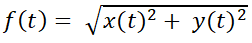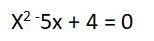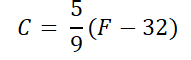# Test Prep Plan - Take a practice test

Take this practice test to check your existing knowledge of the course material. We'll review your answers and create a Test Prep Plan for you based on your results.
How Test Prep Plans work
1
2Based on your results, we'll create a customized Test Prep Plan just for you!
3Study smarter
Study more effectively: skip concepts you already know and focus on what you still need to learn.

# TExES Mathematics/Science 4-8 (114): Practice & Study Guide Final Exam

Free Practice Test Instructions:

Choose your answer to the question and click 'Continue' to see how you did. Then click 'Next Question' to answer the next question. When you have completed the free practice test, click 'View Results' to see your results. Good luck!

#### Question 5 5. Train A and Train B are traveling from the same station at the same time. Train A is traveling due east at a constant rate and has traveled x(t) where train B is traveling due south at a constant rate and has traveled y(t) . The distance between the trains at time t is given by the function below. Which of the following can used to find the instantaneous rate of change between the two trains at time t?#### Question 10 10. Use the equation below to answer the following question.#### Question 13 13. The formula below shows the relationship between C degrees Celsius and F degrees in Fahrenheit. In an experiment, a certain chemical is to be kept in an interval between 15 degrees Celsius and 40 degrees Celsius. What is the temperature interval in degrees Fahrenheit?